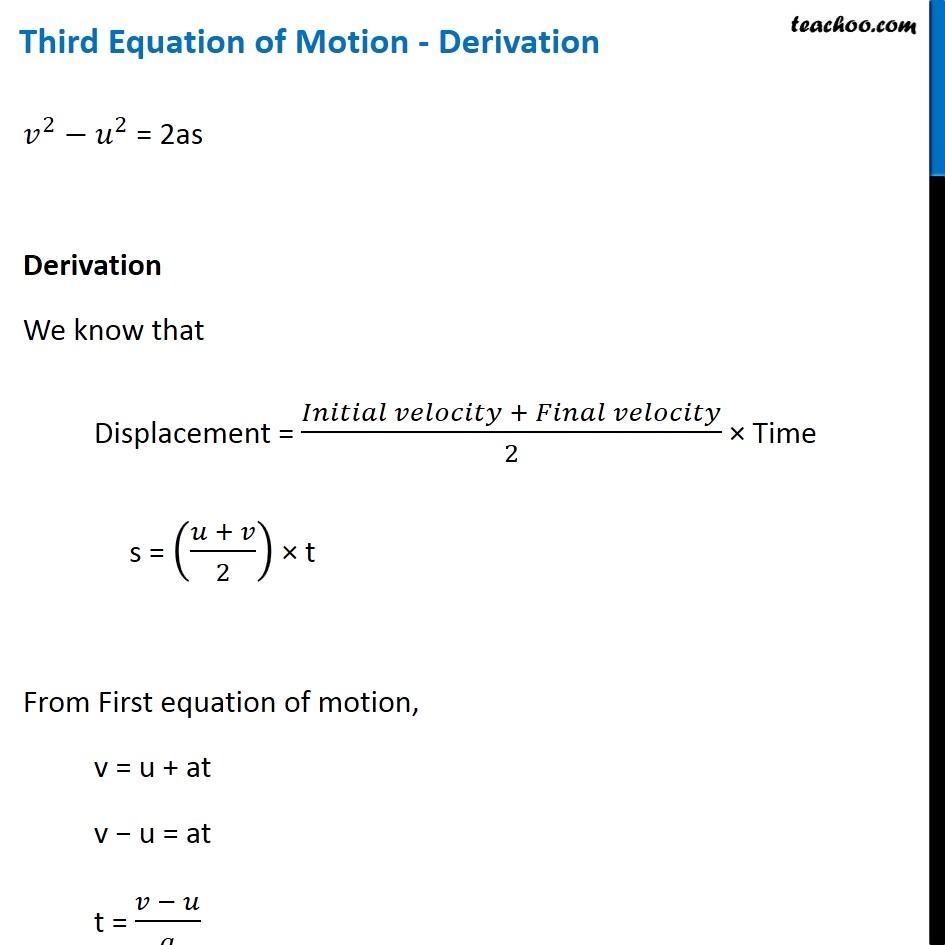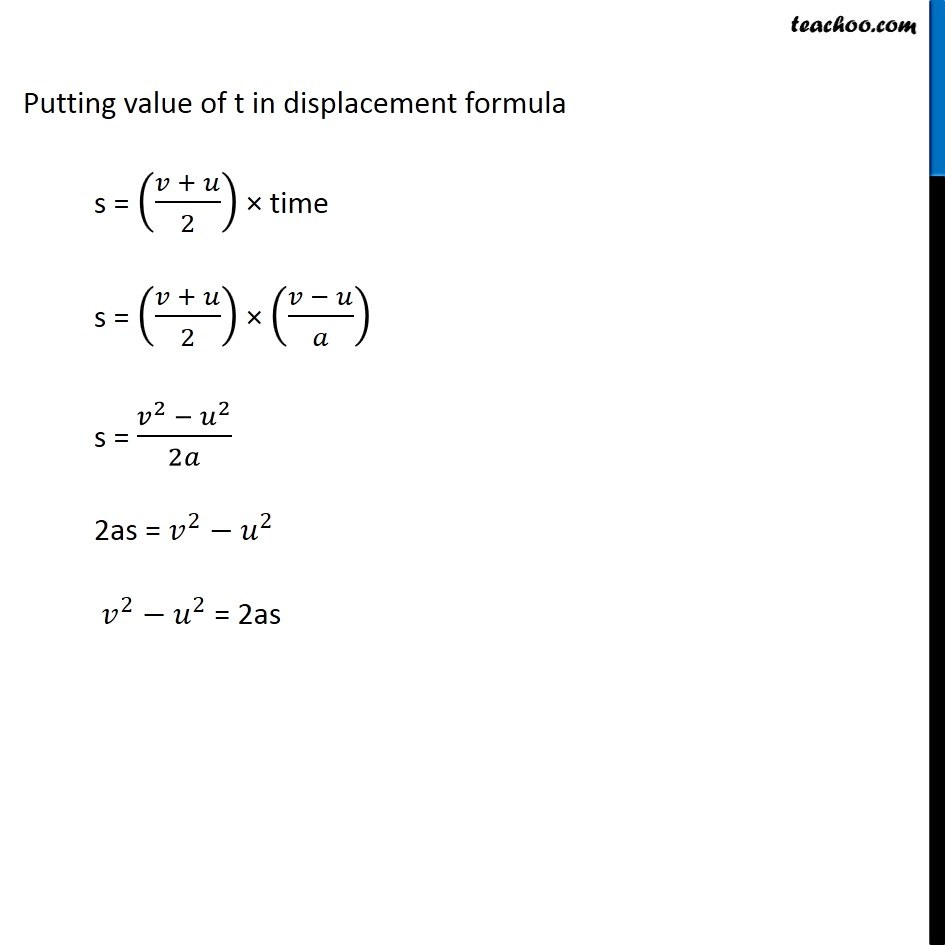Concepts

Class 9
Chapter 8 Class 9 - Motion

it is denoted by

v 2 - u 2 = 2as

Where

v = Final Velocity

u = Initial Velocity

s = Distance Travelled

a = accelerationTo solve problems using equations of motion, we should remember that

• If body starts from rest, its Initial velocity = u = 0
• If we drop a body from some height, its Initial velocity = u = 0
• If body stops, its Final velocity = v = 0
• If body moves with uniform velocity, its Acceleration = a = 0

## Questions

Q 2 Page 110 - A train is traveling at a speed of 90 km h–1. Brakes are applied so as to produce a uniform acceleration of – 0.5 m s-2. Find how far the train will go before it is brought to rest.

Q 5 Page 110 - A stone is thrown in a vertically upward direction with a velocity of 5 m s-1. If the acceleration of the stone during its motion is 10 m s–2 in the downward direction, what will be the height attained by the stone and how much time will it take to reach there?

NCERT Question 7 - A ball is gently dropped from a height of 20 m. If its velocity increases uniformly at the rate of 10 m s-2, with what velocity will it strike the ground? After what time will it strike the ground?

Example 8.5 - A train starting from rest attains a velocity of 72 km h–1 in 5 minutes. Assuming that the acceleration is uniform, find (i) the acceleration and (ii) the distance travelled by the train for attaining this velocity.

Learn in your speed, with individual attention - Teachoo Maths 1-on-1 Class

### Transcript

Third Equation of Motion - Derivation 𝑣^2−𝑢^2 = 2as Derivation We know that Displacement = (𝐼𝑛𝑖𝑡𝑖𝑎𝑙 𝑣𝑒𝑙𝑜𝑐𝑖𝑡𝑦 + 𝐹𝑖𝑛𝑎𝑙 𝑣𝑒𝑙𝑜𝑐𝑖𝑡𝑦)/2 × Time s = ((𝑢 + 𝑣)/2) × t From First equation of motion, v = u + at v − u = at t = (𝑣 − 𝑢)/𝑎 Putting value of t in displacement formula s = ((𝑣 + 𝑢)/2) × time s = ((𝑣 + 𝑢)/2) × ((𝑣 − 𝑢)/𝑎) s = (𝑣^2 − 𝑢^2)/2𝑎 2as = 𝑣^2−𝑢^2 𝑣^2−𝑢^2 = 2as